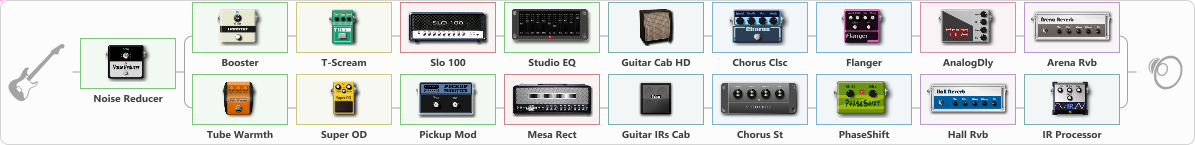# Erect Johnson

Discussion in 'ToneLib-GFX presets' started by davidtaylors, Feb 15, 2022.

1. ### davidtaylorsWell-Known Member

Erect Johnson

Preset name: Erect Johnson

EJ Tone

Effects chain:Effect: "Noise Reducer" (Dynamics / Filter), active - "yes"
{
"Sens" = 63
"Mode" = Hard
}

Effect: "Splitter" (Dynamics / Filter)
{
"A-Bypass" = Off
"A-Pan" = -25
"A-Level" = 55
"B-Bypass" = Off
"B-Pan" = 25
"B-Level" = 55
"Width" = 0

'A' branch:
{

Effect: "Booster" (Dynamics / Filter), active - "yes"
{
"Gain" = 20
}

Effect: "T-Scream" (Overdrive / Distortion), active - "yes"
{
"Drive" = 81
"Tone" = 56
"Level" = 29
}

Effect: "Slo 100" (Amp simulators), active - "yes"
{
"Gain" = 74
"Bass" = 76
"Middle" = 16
"Treble" = 70
"Presence" = 79
"Master" = 50
"Level (dB)" = 0
}

Effect: "Studio EQ" (Dynamics / Filter), active - "yes"
{
"31 Hz" = 0
"62 Hz" = 0
"125 Hz" = 0
"250 Hz" = 0
"500 Hz" = 0
"1 kHz" = 0
"2 kHz" = 0
"4 kHz" = 0
"8 kHz" = 0
"16 kHz" = 0
"Level (dB)" = 0
}

Effect: "Guitar Cab HD" (Cabinets), active - "yes"
{
"Model" = Marshall 1960A (4x12")
"Mic Model" = Dynamic MD421
"Mic Position" = Middle
"Mic Distance" = Middle
"Low Cut (Hz)" = 80
"Hi Cut (kHz)" = 20.0
"Mix" = 100
"Level (dB)" = 0
}

Effect: "Chorus Clsc" (Modulation / Sfx), active - "yes"
{
"Speed" = 3.5
"Depth" = 58
"Center" = 1.0
"Mode" = Stereo
}

Effect: "Flanger" (Modulation / Sfx), active - "yes"
{
"Speed" = 0.8
"Depth" = 35
"Reso" = 35
"Center" = 3.5
"Offset" = 0.0
"Mix" = 54
"Reset" = On
}

Effect: "AnalogDly" (Delay), active - "yes"
{
"Time" = 380
"Feedback" = 34
"Tone" = 65
"Mix" = 65
}

Effect: "Arena Rvb" (Reverberation), active - "yes"
{
"Time" = 7.3
"PreDelay" = 23
"LoDamp" = 18
"HiDamp" = 21
"Mix" = 43
}
}
'B' branch:
{

Effect: "Tube Warmth" (Dynamics / Filter), active - "yes"
{
"Input" = 50
"Warmth" = 50
"Level" = 50
}

Effect: "Super OD" (Overdrive / Distortion), active - "yes"
{
"Drive" = 8
"Tone" = 80
"Level" = 91
}

Effect: "Pickup Mod" (Dynamics / Filter), active - "yes"
{
"Mode" = Phase Out
"Tone" = 50
}

Effect: "Mesa Rect" (Amp simulators), active - "yes"
{
"Gain" = 50
"Bass" = 50
"Middle" = 50
"Treble" = 50
"Presence" = 50
"Master" = 50
"Level (dB)" = 0
}

Effect: "Guitar IRs Cab" (Cabinets), active - "yes"
{
"Model" = Fender Deluxe Rev (1x12")
"Mic Position" = Middle
"Mic Distance" = Near
"Low Cut (Hz)" = 60
"Hi Cut (kHz)" = 20.0
"Mix" = 100
"Level (dB)" = 0
}

Effect: "Chorus St" (Modulation / Sfx), active - "yes"
{
"Speed" = 1.9
"Depth" = 31
"Center" = 4.4
"Mix" = 40
}

Effect: "PhaseShift" (Modulation / Sfx), active - "yes"
{
"Shift" = 73
"Mix" = 43
}

Effect: "Hall Rvb" (Reverberation), active - "yes"
{
"Time" = 6.9
"PreDelay" = 0
"LoDamp" = 0
"HiDamp" = 17
"Mix" = 44
}

Effect: "IR Processor" (Cabinets), active - "yes"
{
"IR" = Rev IR Sample6
"Low Cut (Hz)" = 0
"Hi Cut (kHz)" = 20.0
"Mix" = 100
"Level (dB)" = 0
}
}
}

Note: You will need to download and install the ToneLib-GFX software to use the preset.

#### Attached Files:

• ###### Erect_Johnson.tlgfx
File size:
43.7 KB
Views:
1,860
capoman and ppv like this.
2. ooo finally, some erect johnson